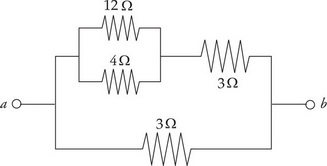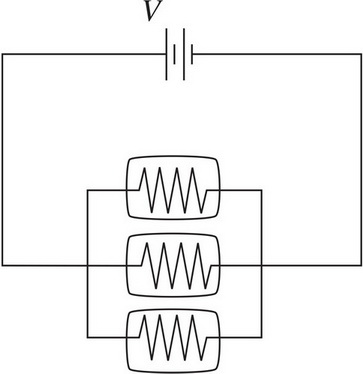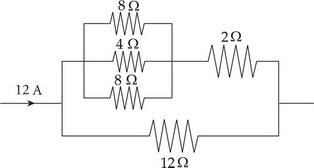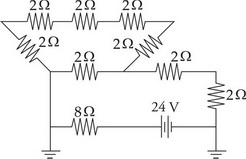# AP Physics 1: Direct Current Circuits Multiple-Choice Practice Questions

### Test Information9 questions18 minutes

1. For an ohmic conductor, doubling the voltage without changing the resistance will cause the current to

2. If a 60-watt light bulb operates at a voltage of 120 V, what is the resistance of the bulb?

3. Which of the following would be most effective at decreasing the resistance of a metal resistor?4. Determine the equivalent resistance between points a and b.5. Three identical light bulbs are connected to a source of emf, as shown in the diagram above. What will happen if the middle bulb burns out?6. What is the voltage drop across the 12 Ω resistor in the portion of the circuit shown above?7. What is the current through the 8 Ω resistor in the circuit shown above?

8. How much energy is dissipated as heat in 20 s by a 100 Ω resistor that carries a current of 0.5 A ?

9. Three light bulbs are initially connected in series to a battery. If a fourth light bulb is then added and is also in series with the other three light bulbs, what happens to the current delivered by the battery?